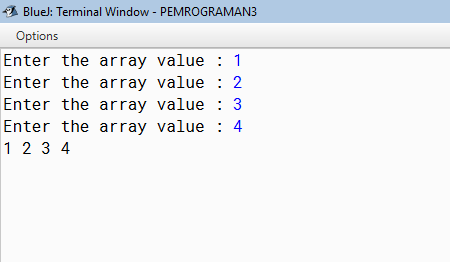Examples of Simple Programs 1 & 2 Dimensional Array in Java

Previously you must have understood what it is to understand the array of variables easier.

So, like this simple example:

When you want to store a data that an instance name, of course, you need to declare a variable to be able to store data such name.

Unfortunately you do not always deal with one data, now you imagine if you want to list the names of students in your class.

Please note: a regular variable can only store one value.

Then, if you will create a variable name1, name2, name3, and so on?

Of course not, this is why you need an array data type. Because of the array allows you to store a lot of data in a variable.

Below is a simple example of the use of java for an array of one-dimensional and two-dimensional array number specified by input.

Example 1 dimensional array java program:

``````import java.util. *;
class input_Array1 {
public static void main (String [] xxx) {
try {
int arr [] = new int ;
String array [] = new String ;
Scanner s = new Scanner (System.in);
for (int i = 0; i <arr.length; i ++) {
System.out.print ("Enter the array value : ");
array [i] = s.next ();
arr [i] = Integer.parseInt (array [i]); // conversion of data types
}
for (int i = 0; i <arr.length; i ++)
System.out.print (arr [i] + " ");
}
catch (Exception e) {
System.err.println (e);
}
}
}
``````the results of coding, variable array will accommodate more than one value

Examples of java program 2 dimensional rows and columns:

``````import java.util. *;
public class ARRAYINPUT_2D {
public static void main (String [] args) {
Scanner scan = new Scanner (System.in);
String [] [] array = new String  ;
for (int i = 0; i <array.length; i ++) {
for (int j = 0; j <array.length; j ++) {
System.out.print ("Input Number line [" + i + "]" + "column [" + j + "] =");
array [i] [j] = scan.next ();
}
System.out.println ("\n");
}
System.out.println ("\n");
System.out.println ("Result of Input Value");
for (int i = 0; i <array.length; i ++) {
for (int j = 0; j <array.length; j ++) {
System.out.print ("|" + array [i] [j] + "|");
}
System.out.println ("");
}
}
}
``````coding results, will be input and display the values ​​of variables array using two-dimensional array in the form of rows and columns

Berlangganan update artikel terbaru via email: